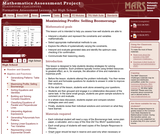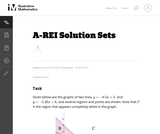Updating search results...

# 5 Results

View
Selected filters:
• CCSS.Math.Content.HSA-REI.D.12Only Sharing Permitted
CC BY-NC-ND
Rating
0.0 stars

This lesson unit is intended to help teachers assess how well students are able to use linear inequalities to create a set of solutions. In particular, the lesson will help teachers identify and assist students who have difficulties in: representing a constraint by shading the correct side of the inequality line; and understanding how combining inequalities affects a solution space.

Subject:
Mathematics
Material Type:
Assessment
Lesson Plan
Provider:
Shell Center for Mathematical Education
Provider Set:
Mathematics Assessment Project (MAP)
04/26/2013Conditional Remix & Share Permitted
CC BY-NC
Rating
0.0 stars

Modeling Our World with Mathematics Unit 1: Health & Fitness Topic 2 - Sports & Fitness

Subject:
Mathematics
Material Type:
Module
Author:
Hannah Hynes-Petty
Washington OSPI Mathematics Department
Washington OSPI OER Project
09/29/2020Unrestricted Use
CC BY
Rating
0.0 stars

This task is the last in a series of three tasks that use inequalities in the same context at increasing complexity in 6th grade, 7th grade and in HS algebra. Students write and solve inequalities, and represent the solutions graphically. The progression of the content standards is 6.EE.8 to 7.EE.4 to A-REI.12.

Subject:
Mathematics
Algebra
Material Type:
Activity/Lab
Provider:
Illustrative Mathematics
Provider Set:
Illustrative Mathematics
Author:
Illustrative Mathematics
05/01/2012Only Sharing Permitted
CC BY-NC-ND
Rating
0.0 stars

This lesson unit is intended to help teachers assess how well students are able to: interpret a situation and represent the constraints and variables mathematically; select appropriate mathematical methods to use; explore the effects of systematically varying the constraints; interpret and evaluate the data generated and identify the optimum case, checking it for confirmation; and communicate their reasoning clearly.

Subject:
Measurement and Data
Material Type:
Assessment
Lesson Plan
Provider:
Shell Center for Mathematical Education
Provider Set:
Mathematics Assessment Project (MAP)
04/26/2013Unrestricted Use
CC BY
Rating
0.0 stars

The typical system of equations or inequalities problem gives the system and asks for the graph of the solution. This task turns the problem around. It gives a solution set and asks for the system that corresponds to it. The purpose of this task is to give students a chance to go beyond the typical problem and make the connections between points in the coordinate plane and solutions to inequalities and equations. Students have to focus on what the graph is showing.

Subject:
Mathematics
Algebra
Material Type:
Activity/Lab
Provider:
Illustrative Mathematics
Provider Set:
Illustrative Mathematics
Author:
Illustrative Mathematics